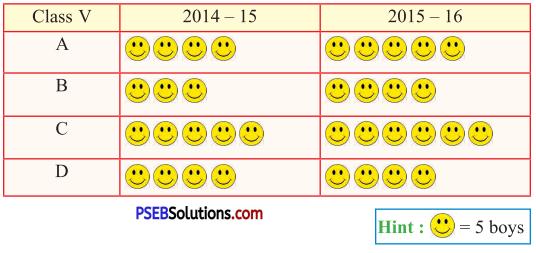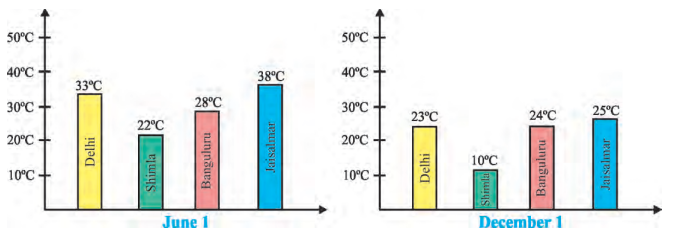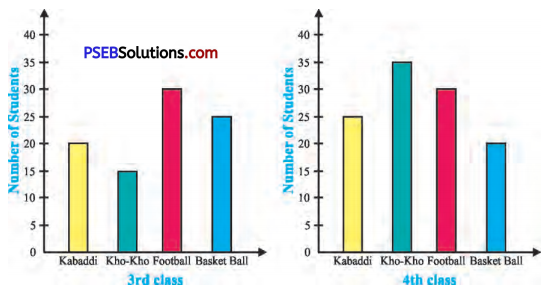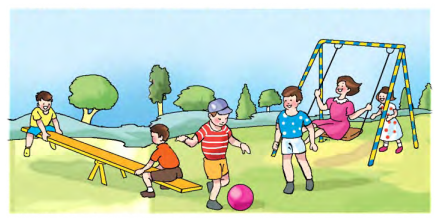# PSEB 5th Class Maths Solutions Chapter 10 Data Handling Ex 10.2

Punjab State Board PSEB 5th Class Maths Book Solutions Chapter 10 Data Handling Ex 10.2 Textbook Exercise Questions and Answers.

## PSEB Solutions for Class 5 Maths Chapter 10 Data Handling Ex 10.2

1. The following pictograph represents the number of boys of sections A, B, C, D of 5th class during sessions 2014-15 and 2015-16.Question 1.
In which session number of boys are more in Section A.
Solution:
2015-16

Question 2.
The number of students of Section D are ……………… in 2014-15 and 2015-16 (equal/more/less)
Solution:
equalQuestion 3.
Find the total number of boys in 2014-15.
Solution:
16 × 5 = 80

Question 4.
Find the difference in number of boys of section C during both sessions.
Solution:
30 – 25 = 5

Question 5.
How many more/less boys are there in 2015-16 than in 2014-15.
Solution:
2015 – 16, 15 more boys.

2. The following two Bar Graphs represent the maximum temperature of four cities on two different days. Four cities are Delhi, Shimla, Bangluru and Jaisalmer.Answer the following from above Bar Graphs :
Question 1.
Which city is the hottest on June 1 ?
Solution:
JaisalmerQuestion 2.
Which city is the coldest on December 1 ?
Solution:
Shimla

Question 3.
What is the difference in Delhi’s temperature on June 1 and December 1 ?
Solution:
33°C – 23° C = 10°C

Question 4.
What is die difference in Shimla’s temperature on June 1 and December 1 ?
Solution:
22°C – 10° C

Question 5.
Which city has lowest change in temperature on June 1 and December 1 ?
Solution:
Bangluru

3. Study the following Bar Graph carefully.Find :
Question 1.
What information is provided by the above Bar Graphs ?
Solution:
The Bar Graphs give us the information about the games played by the students of class 3rd and 4th and the number of students who play that game.Question 2.
Which game is played equally by students of 3rd and 4th class ?
Solution:
Football

Question 3.
What is the total number of students of 3rd and 4th class who play Kho- Kho?Solution:
15 + 35 = 50

Question 4.
What is the total number of students Of 3rd and 4th class who play Basketball ?
Solution:
25 + 20 = 45

Question 5.
Which is the favourite game of 3rd class students ?
Solution:
FootballQuestion 6.
Which is the favourite game of 4th class students ?
Solution:
Kho-Kho.